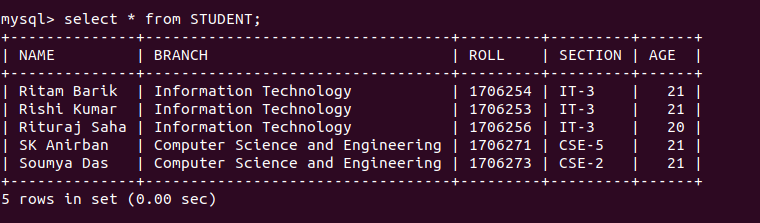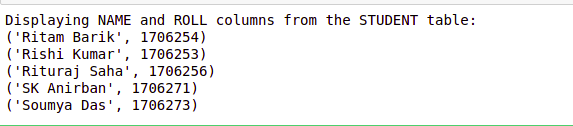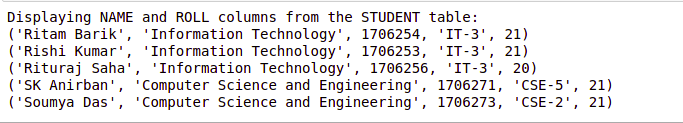Related Articles
Python MySQL – Select Query
• Last Updated : 06 Mar, 2020

Python Database API ( Application Program Interface ) is the Database interface for the standard Python. This standard is adhered to by most Python Database interfaces. There are various Database servers supported by Python Database such as MySQL, GadFly, mSQL, PostgreSQL, Microsoft SQL Server 2000, Informix, Interbase, Oracle, Sybase etc. To connect with MySQL database server from Python, we need to import the `mysql.connector` module.

Below is a program to connect with MySQL database `geeks`.

 `# importing required library ``import` `mysql.connector`` ` `# connecting to the database ``dataBase ``=` `mysql.connector.connect(``                     ``host ``=` `"localhost"``,``                     ``user ``=` `"user"``,``                     ``passwd ``=` `"pswrd"``,``                     ``database ``=` `"geeks"` `) `` ` `# preparing a cursor object ``cursorObject ``=` `dataBase.cursor() `` ` `# disconnecting from server``dataBase.close() `

The above program illustrates the connection with the MySQL database `geeks` in which host-name is `localhost`, the username is `user` and password is `pswrd`.

## Select Query

After connecting with the database in MySQL we can select queries from the tables in it.

Syntax:

• In order to select particular attribute columns from a table, we write the attribute names.
`SELECT attr1, attr2 FROM table_name`
• In order to select all the attribute columns from a table, we use the asterisk ‘*’ symbol.
`SELECT * FROM table_name`

Example 1: Let’s consider the table looks like this –Below is a program to select a query from the table in the database.

 `# importing required library ``import` `mysql.connector ``   ` `# connecting to the database ``dataBase ``=` `mysql.connector.connect(``                     ``host ``=` `"localhost"``,``                     ``user ``=` `"user"``,``                     ``passwd ``=` `"pswrd"``,``                     ``database ``=` `"geeks"` `) ``   ` `# preparing a cursor object ``cursorObject ``=` `dataBase.cursor()``   ` `print``(``"Displaying NAME and ROLL columns from the STUDENT table:"``)`` ` `# selecting query``query ``=` `"SELECT NAME, ROLL FROM STUDENT"``cursorObject.execute(query)`` ` `myresult ``=` `cursorObject.fetchall()`` ` `for` `x ``in` `myresult:``    ``print``(x)`` ` `# disconnecting from server``dataBase.close()`

Output:Example 2: Let us look at another example for selecting queries in a table.

 `# importing required library ``import` `mysql.connector ``   ` `# connecting to the database ``dataBase ``=` `mysql.connector.connect(``                     ``host ``=` `"localhost"``,``                     ``user ``=` `"user"``,``                     ``passwd ``=` `"pswrd"``,``                     ``database ``=` `"geeks"` `)  ``   ` `# preparing a cursor object ``cursorObject ``=` `dataBase.cursor()``   ` `print``(``"Displaying NAME and ROLL columns from the STUDENT table:"``)`` ` `# selecting query``query ``=` `"SELECT * FROM STUDENT"``cursorObject.execute(query)`` ` `myresult ``=` `cursorObject.fetchall()`` ` `for` `x ``in` `myresult:``    ``print``(x)`` ` `# disconnecting from server``dataBase.close()`

Output:Attention geek! Strengthen your foundations with the Python Programming Foundation Course and learn the basics.

To begin with, your interview preparations Enhance your Data Structures concepts with the Python DS Course.

My Personal Notes arrow_drop_up